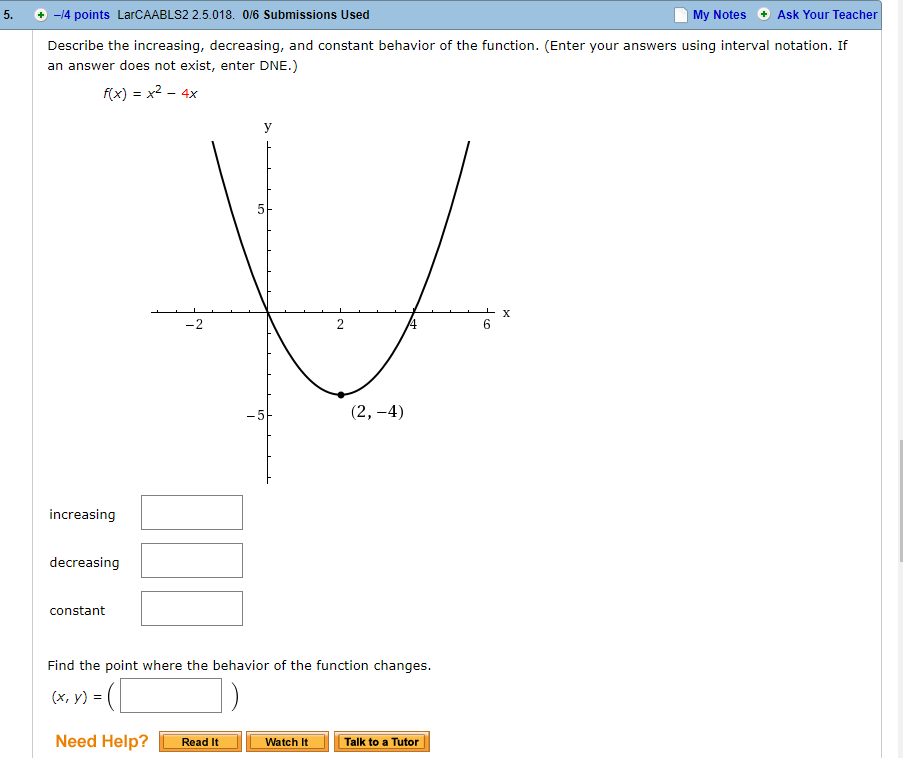# 5. +-/4 points LarCAABLS2 2.5.018. 0/6 Submissions Used My Notes Ask Your Teacher Describe the increasing, decreasing, and constant behavior of the function. (Enter your answers using interval notation. If an answer does not exist, enter DNE.) f(x) = x2-4x 6 increasing decreasing constant Find the point where the behavior of the function changes. (x, y) = Need Help? LlReadIt Watch It Talk to a Tutor

Question

5help_outlineImage Transcriptionclose5. +-/4 points LarCAABLS2 2.5.018. 0/6 Submissions Used My Notes Ask Your Teacher Describe the increasing, decreasing, and constant behavior of the function. (Enter your answers using interval notation. If an answer does not exist, enter DNE.) f(x) = x2-4x 6 increasing decreasing constant Find the point where the behavior of the function changes. (x, y) = Need Help? LlReadIt Watch It Talk to a Tutor fullscreen